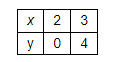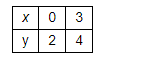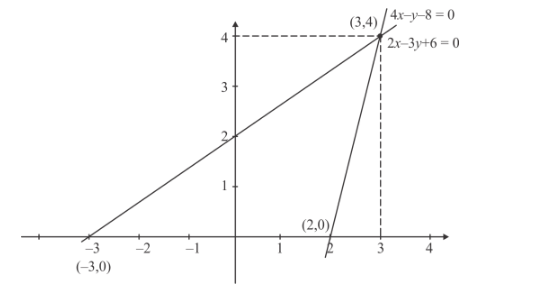# Check graphically whether the pair of linear equation`
Question:

Check graphically whether the pair of linear equation 4x − y − 8 = 0 and 2x − 3y +  6 = 0 is consistent. Also, find the vertices of the triangle formed by these lines with the x-axis.

Solution:

Here we have to draw the graph between two equations given by

$4 x-y-8=0$.........(1)

$2 x-3 y+6=0$............(2)

Also we have to find the vertices of the triangle form by the x-axis and these lines.

The first equation can written as follow

$y=4 x-8$ ............(3)

Now we are going to find the value of y at different value of xNow mark the points (0,-8) and (2, 0) on xy −plane and we will draw a line which passes through these two points.

The second equation can be written as

$y=\frac{2 x+6}{3}$.............(4)Now mark the points (0, 2) and (3, 4) on xy −plane and we will draw a line which passes through these two points.

From the above analysis, we have the following graphFrom the above graph the vertices AB and C are given as follow.

$A=(3,4)$

$B=(2,0)$

$C=(-3,0)$

Hence the vertices are $(3,4),(2,0)(-3,0)$